# Test: AC Voltage Controllers

## 30 Questions MCQ Test Power Electronics | Test: AC Voltage Controllers

Description
Attempt Test: AC Voltage Controllers | 30 questions in 25 minutes | Mock test for Electrical Engineering (EE) preparation | Free important questions MCQ to study Power Electronics for Electrical Engineering (EE) Exam | Download free PDF with solutions
QUESTION: 1

### AC voltage controllers convert

Solution:

Voltage controllers convert the fixed ac voltage to variable ac by changing the values of the firing angle.

QUESTION: 2

### In AC voltage controllers the

Solution:

Voltage controllers convert the fixed ac voltage to variable ac by changing the values of the firing angle. The available ac obtained has the same fixed frequency as the input ac.

QUESTION: 3

### Earlier then the semiconductor technology, ___________ devices were used for voltage control applications.

Solution:

A tap changing transformer can give variable ac from fixed ac without a change in frequency.

QUESTION: 4

The AC voltage controllers are used in __________ applications.

Solution:

In electric heating, variable ac supply is needed. The devices are fired appropriately to apply enough temperature.

QUESTION: 5

In the principle of phase control

Solution:

Switching devices is so operated that the load gets connected to ac source for a part of each half cycle.

QUESTION: 6

A single-phase half wave voltage controller consists of

Solution:

As it is half wave, it consists of one SCR ( the control element) in anti parallel with one diode.

QUESTION: 7

In the below given voltage controller circuit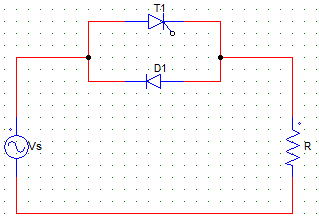Solution:

As T1 is triggered at an angle α, the conduction in the positive have cycle will start at α. In the negative half cycle, the diode is forward biased and load is connected as it is to the supply.

QUESTION: 8

The below shown controller circuit is a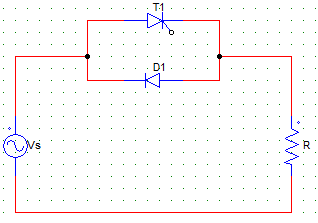Solution:

As it consists one diode and one SCR only, the control is only in one cycle (the positive half in this case), hence it is a half wave controller.

QUESTION: 9

The below given controller circuit is a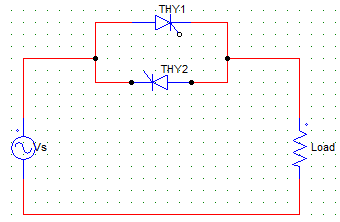Solution:

As it consists of two SCRs, it is a full wave controller.

QUESTION: 10

In the below given voltage controller circuit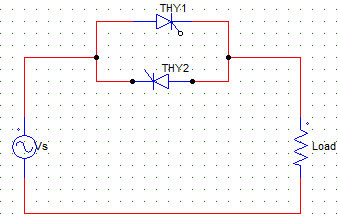Solution:

As it consists of two SCRs, it is a full wave controller and both the half cycles can be controlled by varying their respective firing angles.

QUESTION: 11

In the below shown circuit, the diode conducts for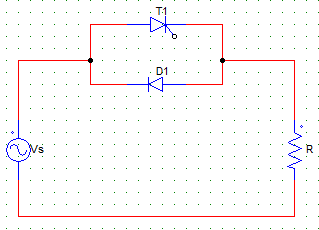Solution:

The diode conducts from π to 2π in the negative half cycle. If it were a non resistive load, the diode would conduct for less than 90°, as the inductor would force conduct the SCR for some time.

QUESTION: 12

The SCR T1 is fired at an angle of α, and the supply Vs = Vm sinωt. Find the average value of the output voltage.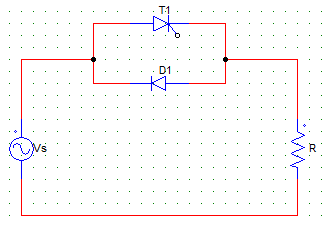Solution:

Vo = (1/2π) ∫Vm sinωt d(ωt). Where the integration would be run from α to 2π as the conduction takes place from α to 2π.
Vo = (Vm/2π) (cosα – 1).

QUESTION: 13

The below given output voltage waveform can be obtained by a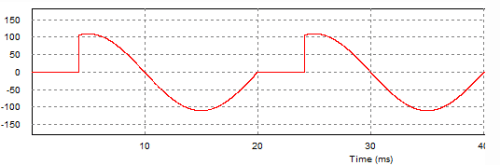Solution:

As the positive half is chopped off due to some value of firing angle, the firing angle is not equal to zero. As the negative half is a half sine wave, either it is a full wave controller with firing angle for T2 set to zero or it is a half wave controller with a Thyristor (T1) and a diode.

QUESTION: 14

In the below shown AC converter circuit with firing angle = α for both the devices, T2 will conduct from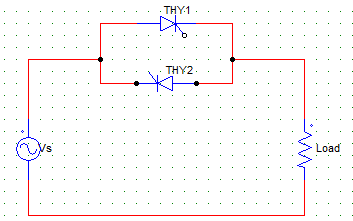Solution:

T2 is triggered at π + α, it conducts from π + α to 2π after which it is line commutated.

QUESTION: 15

The below given output voltage waveform can be obtained by a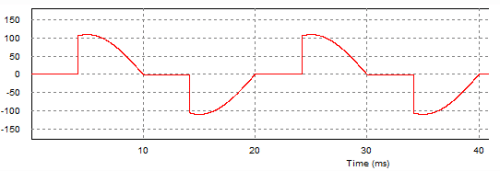Solution:

As the control is in both the directions or cycles, it is a full wave ac voltage controller circuit.

QUESTION: 16

In the integral cycle control method of ac voltage controller

Solution:

In integral cycle control, power is delivered for m cycles and not delivered for n cycles. Hence, the average value of the power delivered is controlled by manipulating m and n.

QUESTION: 17

In the integral cycle control of ac voltage controller, is the load is on for n cycles and off for m cycles, then the periodicity is given by? Consider the output is sinusoidal.

Solution:

Over a complete cycle of 2π x (on cycles + off cycles) the power is delivered for n cycles.
Hence, P = n/2π(m+n).

QUESTION: 18

If k is the duty cycles of the controller, then the rms value of the output voltage in case of a integral cycle control circuit will be?
Consider the input to be sinusoidal with peak value Vm and rms value Vs.

Solution:

Vrms = [ (k/2π) x ∫Vmsin⁡2 ωt d(ωt) ] 1/2
Where the integral runs from 0 to 2π.
Vrms = (Vm/√2) k = Vs x √k.

QUESTION: 19

Find the power delivered to the load in the integral cycle control method of ac voltage control, having a sine input of Vs, R load and duty cycle = k.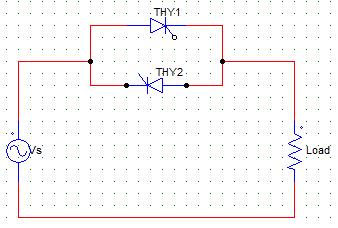Solution:

Output voltage = Vs x √k
P = (Vs x √k)2/R.

QUESTION: 20

In the integral cycle control method with duty cycle = k and maximum load current = Im. Find the value of average SCR current.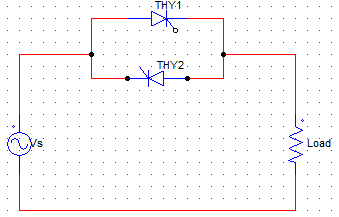Solution:

As each SCR conducts for π radians during each cycle of n on cycles, the average value of SCR current is
I = (k/2π) ∫Im sin ωt d(ωt). Where the integration is from 0 to π.

QUESTION: 21

The below given circuit has Vs = 230V and R = 20 Ω. Find the value of the average output voltage at the R load for a firing angle of 45°.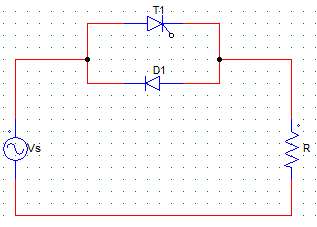Solution:

Vo = [(√2 x 230) x (cos45 – 1)]/2π = -15.17 V.
Negative value is due to the fact that the average value in the positive half cycle is less than that in the negative half cycle.

QUESTION: 22

The below given circuit has Vs = 230V and R = 20 Ω. Find the value of the average output load current at the R load for a firing angle of 45°.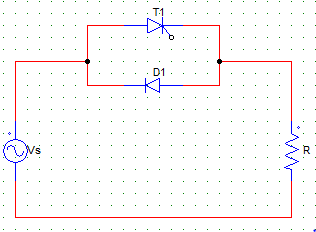Solution:

Vo = [(√2 x 230) x (cos45 – 1)]/2π = -15.17 V.
Io = (Vo)/R = -0.7585
Negative value is due to the fact that the average value in the positive half cycle is less than that in the negative half cycle.

QUESTION: 23

A single phase voltage controller has input of 230 V and a load of 15 Ω resistive. For 6 cycles on and 4 cycles off, determine the rms output voltage.

Solution:

Vrms = Vo x √k
k = (6/6+4) = 6/10 = 0.6
Vrms = √0.6 x 230 = 178.157 V.

QUESTION: 24

A single phase voltage controller has input of 230 V and a load of 15 Ω resistive. For 6 cycles on and 4 cycles off, determine the input pf.

Solution:

k = (6/6+4) = 6/10 = 0.6
input pf = √0.6 = 0.7746.

QUESTION: 25

A single phase voltage controller has input of 230 V and a load of 15 Ω resistive. For 6 cycles on and 4 cycles off, determine the power delivered to the load.

Solution:

Vrms = Vo x √k
k = (6/6+4) = 6/10 = 0.6
Vrms = √0.6 x 230 = 178.157 V
P = (Vrms)2/R = 2116 W = 2.116 kW.

QUESTION: 26

A single phase voltage controller has input of 230 V and a load of 15 Ω resistive. For 6 cycles on and 4 cycles off, determine the average value of SCR current.

Solution:

Peak current Im = (230 x √2)/15 = 21.681 A
k = (6/6+4) = 6/10 = 0.6
Avg current = (k Im)/π = 4.14 A.

QUESTION: 27

Pulse gating is suitable for

Solution:

In RL loads with pulse gating, the incoming SCR may be fired during the interval when it is reversed biased by the outgoing SCR, thus it won’t get turn on even after the outgoing SCR is not reveres basing the incoming SCR is the pulse width is over before forward biasing the SCR.

QUESTION: 28

In continues gating

Solution:

As the gating is applied for a longer duration, the device is heated up.

QUESTION: 29

High frequency gating uses a

Solution:

In high frequency gating a train of pulses are used to overcome the thermal problems due to continuous gating.

QUESTION: 30

A single-phase voltage controller, using one SCR in anti parallel with a diode, feeds a load R and Vs = 230 V. For a firing angel of 90° for the SCR, the PMMC voltage connected across R would read

Solution:

As firing angle is 90, there is ideally be no conduction in the positive half. Hence, the average value will be zero.
Vo = (√2 Vs)/2π x (cos90 – 1) = – 51.8 V.Use Code STAYHOME200 and get INR 200 additional OFF Use Coupon Code